# Series LC circuit producing amplitude modulation?

#### electronice123

Joined Oct 10, 2008
335
Hello all,

Using multisim to simulate a series LC circuit I am seeing amplitude modulation across the capacitor at the resonant freq. I don't understand why this is happening. I am using the ac power supply with a 1.5H inductor and a 300pF capacitor in series. I have the oscilloscope placed across the capacitor and at the resonant frequency I get amplitude modulation. My question is why?
Shouldn't I just be seeing AC at a higher voltage since its at resonance? What is causing the amplitude modulation?

#### crutschow

Joined Mar 14, 2008
32,938

#### electronice123

Joined Oct 10, 2008
335
Will do, I'll have to wait till I get my computer though.

#### electronice123

Joined Oct 10, 2008
335
Here's the schematic on multisim along with the oscilloscope traces (both taken across the capacitor). Even when I remove the parallel capacitance the circuit produces AM...
Why is the circuit producing Amplitude modulation?

#### nigelwright7557

Joined May 10, 2008
532
Here's the schematic on multisim along with the oscilloscope traces (both taken across the capacitor). Even when I remove the parallel capacitance the circuit produces AM...
Why is the circuit producing Amplitude modulation?
It shouldnt produce AM. Remove the capacitor in parallel.
You have a series LC so above and below resonance it should have an output.
At resonance you will get a very low output due to resistance in circuit.

#### crutschow

Joined Mar 14, 2008
32,938
That's an interesting response. It appears to be a beat frequency between the actual resonant frequency of the circuit and the input signal frequency due to the extremely high Q of that ideal circuit (no resistance in the circuit). A change in the input frequency changes the beat frequency. And if you simulate for a half second or so, the beat frequency damps out. An input frequency of about 7390Hz seems to generate an amplitude increasing without end in my (LTspice) simulation (up to hundreds of kV output at 2 seconds) with no modulation so that would appear to be close to the actual resonant frequency of the circuit.

You can cause the circuit to settle out much faster if you add some reasonable series resistance to the inductor. For example, 1k ohm cause the circuit to settle in about 10ms.

All this is a reminder that you can get some strange (but not incorrect) results when simulating ideal circuits.

#### MrAl

Joined Jun 17, 2014
10,621
Hi,

Yes it is not really amplitude modulation it is a beat frequency between the natural frequency of the network and the input frequency. That means that as time runs on, sometimes the two are adding and sometimes they subtract which makes hills and valleys over time.

To see how this works exactly, a representative function for the response of this network with the given values (source amplitude is equal to 1v peak though) is:
Vc2(t)=((w^2-1.3333333328899998*10^11)*cos(w*t)+1.31147540907*10^11*cos(46752.45833333334*t))/(61*w^2-1.3333333328899998*10^11)

where w is 2*pi*f and f is the frequency of the source (a cosine source is used here rather than a sine source but the response after a decent amount of time will look the same except for the phase).

What we see here is one cosine response that has fixed frequency (on the right) and one that is variable and the variable one is the driving voltage source. The two either add or subtract depending on the point in time, except for one very special frequency, and that is the frequency that forces the denominator to zero, and when that happens the function breaks down into a simpler function that gives us a simple response:
Vc2(t)=0.0163934426*cos(46752.45833333334*t)

however this only happens when the source frequency is the exact value as explained above and this is mainly a theoretical point as it would be hard to produce in real life or even in a simulator. So in theory the beat only happens when the source frequency is not the right value, but unfortunately that will be (except in theory) almost alwaysIt should also be added that it may take some time to see the beat mix because it depends how much the two frequencies are out of sync.
Also note that Vc2(t) given here will be about 1/14 that of the circuit given because the source here is only 1v peak not 10v rms. The results should scale just fine however.

Last edited:

#### The Electrician

Joined Oct 9, 2007
2,936
... I am seeing amplitude modulation across the capacitor at the resonant freq
What you're seeing isn't what "ordinary" amplitude modulation looks like.
For example, here's what 50% AM looks like: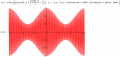Here's what 100% AM looks like: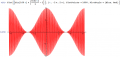But, now let's begin to suppress the carrier. Here's what it looks like with only half the carrier: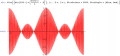Now with only 1/4 the carrier: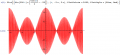Finally, with the carrier completely suppresed. This is double sideband, suppressed carrier: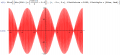The waveform you're seeing is like double sideband, suppressed carrier, but it isn't produced by a modulation process like the one just above.

Remember the trigonometric identity:

$$Sin(x)+Sin(y) = 2 Sin(\frac{x+y}{2})*Sin(\frac{x-y}{2})$$

The sum of two sine waves very close together in frequency can produce a waveform which is also the product of two sine waves. It's that product that is equivalent to modulation.

Here's what it looks like when we add two sine waves of radian frequencies 99 and 101. It's identical to the waveform produced by amplitude modulation with a suppressed carrier just above: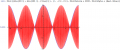When you run the simulation, you are suddenly applying a driving signal to your circuit. That causes the circuit to "ring" at its natural resonance frequency. Normally it would die out in a while due to losses in the circuit, but your simulation includes no losses, so the "ringing" continues for quite a while. If the natural "ring" frequency is very close to the driving frequency, then your circuit has two sine waves added together which are nearly the same frequency. That gives you a result like that just above.

It doesn't look like "ordinary" amplitude modulation, but it does look like double sideband suppressed carrier. Such a waveform can be produced by a modulation process, but it can also be produced by just the addition of two sine waves of nearly the same frequency. In your circuit, there is no modulation, just simple addition of sine waves.

•MrAl

#### electronice123

Joined Oct 10, 2008
335
Wow, great responses. Thank you all very much.

#### MrAl

Joined Jun 17, 2014
10,621
Hi again,

I am not easily impressed by replies (although i still enjoy most of them) but i have to say i was very impressed by Electricians post #8 which clearly shows many different types of waveforms and what makes them appear like that. Seeing the waveforms helps understanding greatly. I was going to post a couple waves too but got a little lazy so thanks to Electrician for that post.